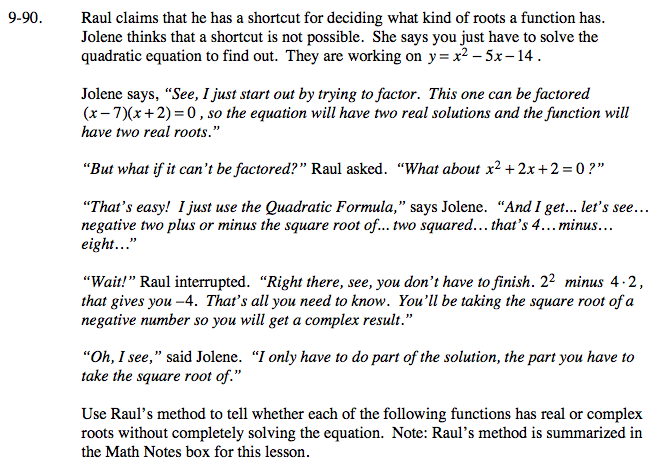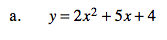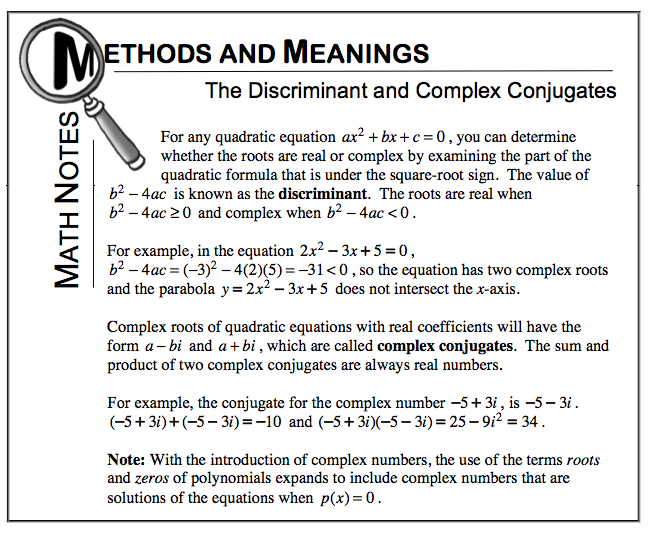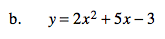Home > A2C > Chapter 9 > Lesson 9.2.2 > Problem9-90

9-90.
1. Raul claims that he has a shortcut for deciding what kind of roots a function has. Jolene thinks that a shortcut is not possible. She says you just have to solve the quadratic equation to find out. They are working on y = x2 − 5x − 14.

2. Use Raul's method to tell whether each of the following functions has real or complex roots without completely solving the equation. Note: Raul's method is summarized in the Math Notes box for this lesson. Homework Help ✎

1. y = 2x2 + 5x + 4

2. y = 2x2 + 5x − 3For the following, refer to the Math Note above from Lesson 9.2.2 (pg 460 in the student textbook).

b2 − 4ac = −7; complex rootsb2 − 4ac = 49
Are the roots real or complex?